# How to List Only Whole Numbers From Decimal Numbers in Excel

This post will guide you how to filter only whole numbers from decimal numbers in a list in Excel. How do I filter cells with whole numbers or non-whole numbers in Excel.

## List Only Whole Numbers

Assuming that you have a list of data in range A1:A6, which contain decimal numbers and whole numbers. And you want to filter only the whole numbers or all non-whole numbers from the given list of data in Excel. You need to create a helper column next to the given data or range, and then using a formula based on INT function to check if the given number is integer or not. If return True, it indicates that this number is an integer or whole number. Otherwise, it is a non-whole number.  Do the following steps:

#1 create a new column next to the numbers column, and type the following formula into cell B1.

=INT(A2)=A2

#2 press Enter key to apply this formula, and drag the AutoFill Handle down to other cells to apply this formula.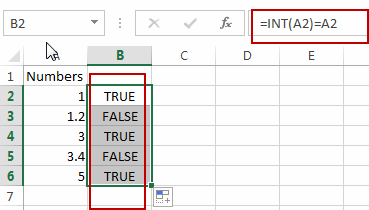#3 select the helper column B, and go to DATA tab, click Filter button under Sort & Filter group. And one Filter icon added into the first cell in helper column.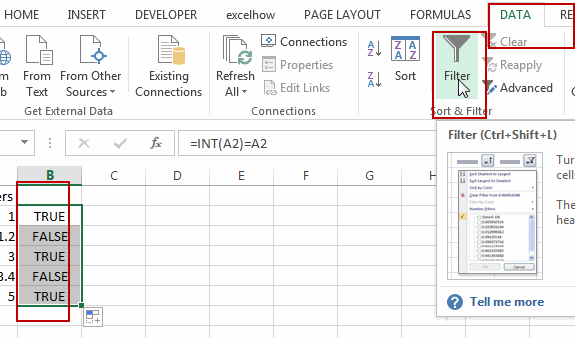#4 click filter icon in the Cell B1, and select TRUE or FALSE value as you what to filter out whole numbers or non-whole numbers. Click Ok button to apply for changes.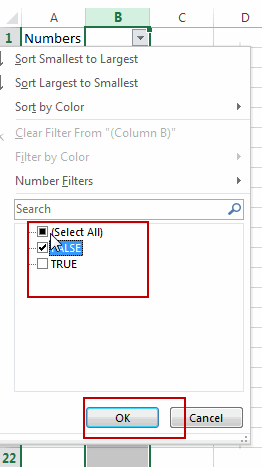#5 you should see that all whole numbers have been filtered out . And if select FALSE option from the drop down list, and it will filtered out all decimal numbers.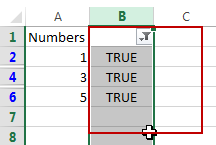Note: you can also use another formula based on the IF function and the MOD function to achieve the same result. Like this:

=IF(MOD(A1,1)<>0,0,A1)

Type this formula into Cell C2 in a help column, and press Enter key.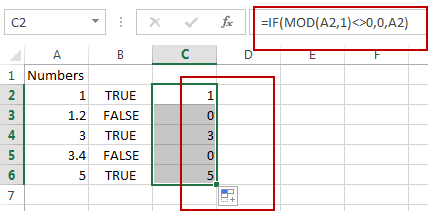You should see that all whole numbers have been listed. And all decimal number will be shown as 0.

### Related Functions

• Excel INT function
The Excel INT function returns the integer portion of a given number. And it will rounds a given number down to the nearest integer.The syntax of the INT function is as below:= INT (number)…
Related Posts

Calculate Number of Hours between Two Times

Calculating the difference between two times might be a valuable statistic for subsequent computations or averages, whether you're producing a time sheet for staff or recording personal exercises. While Excel has a plethora of complex functions, including date and time ...

Calculate Grades With VLookup in Excel

Why Should You Calculate Grades With VLookup Excel? If you're looking for a simple way to find out a student's grades, VLOOKUP Excel can do the trick. This function uses a lookup table to find the values and sort them ...

Extract date from multiple cells containing both date and time

As if you have got a cell containing date along with the time in the same cell and you want to extract the date into a separate cell, then for one or two cells, you can extract the date manually ...

How to Auto Fill Weekdays or Weekends in Excel

Sometimes we may want to enter a sequence of days in excel for some purpose, and we can press Ctrl+; to insert current date into cell, and then drag the cell down to attach following days into other cells, then ...

How to Stack Data from Multiple Columns into One Column in Excel

In previous article, I have shown you the method to split data from one long column to multiple columns by VBA and Index function. This time if we want to stack data from multiple columns to one column, how can ...

How to Calculate Retirement Date and Remaining Years refer to Birth Date in Excel

Every company has its own police about the retirement date for employees. Suppose employees will be retired from a certain age of 60, how can we calculate the retirement date for everyone base on their birthdays? Actually, there are several ...

How to Calculate Number of Weekends between Two Dates in Excel

This post will guide you how to count the number of weekends between two given dates in Excel. How to find out how many weekends are between two dates in Excel. How to count the number of Sundays between two ...

How to Check If a Number is Integer in Excel

This post will guide you how to check if a number is integer in Cells in Excel. How do I test if Cell value is integer with a formula in Excel. Check If Value is Integer Assuming that you have ...

How to Delete Numbers after Decimal Point in Excel

This post will guide you how to remove decimals without rounding in Excel. How do I delete numbers after decimal point with a formula in the given cell in Excel. Deleting Numbers after Decimal Point Assuming that you have a ...

Count the Number of Weekends between Two Date

This post will guide you how to count the number of weekends between two dates in Excel. How do I get the number of weekends between 2 dates with a formula in Excel. How to count weekend days between dates ...

Sidebar